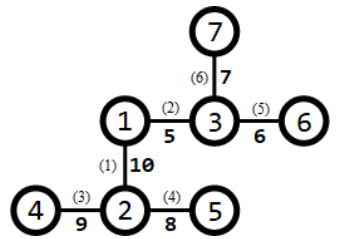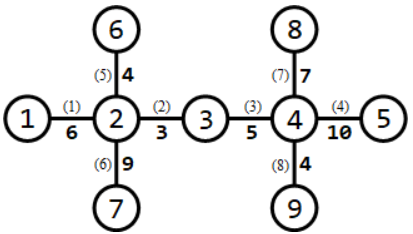# #438. 【NOIP2018】赛道修建

C 城将要举办一系列的赛车比赛。在比赛前，需要在城内修建 $m$ 条赛道。

C 城一共有 $n$ 个路口，这些路口编号为 $1,2, \cdots , n$，有 $n − 1$ 条适合于修建赛道的双向通行的道路，每条道路连接着两个路口。其中，第 $i$ 条道路连接的两个路口编号为 $a_i$ 和 $b_i$，该道路的长度为 $l_i$。借助这 $n − 1$ 条道路，从任何一个路口出发都能到达其他所有的路口。

### 样例一

#### input

7 1
1 2 10
1 3 5
2 4 9
2 5 8
3 6 6
3 7 7

#### output

31

#### 样例解释 1### 样例二

#### input

9 3
1 2 6
2 3 3
3 4 5
4 5 10
6 2 4
7 2 9
8 4 7
9 4 4

#### output

15

#### 样例解释 2### 限制与约定

$1$ $\le 5$ $=1$
$2$ $\le 10$ $\le n-1$
$3$ $\le 15$
$4$ $\le 10^3$ $=1$
$5$ $\le 3\times 10^4$
$6$
$7$ $\le n-1$
$8$ $\le 5\times 10^4$
$9$ $\le 10^3$
$10$ $\le 3\times 10^4$
$11$ $\le 5\times 10^4$
$12$ $\le 50$
$13$
$14$ $\le 200$
$15$
$16$ $\le 10^3$
$17$
$18$ $\le 3\times 10^4$
$19$
$20$ $\le 5\times 10^4$